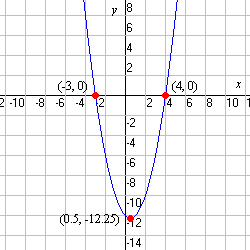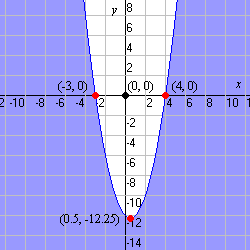A quadratic inequality of the form

$y>a{x}^{2}+bx+c$

(or substitute $<,\ge$ or $\le$ for $>$ ) represents a region of the plane bounded by a parabola .

To graph a quadratic inequality, start by graphing the parabola. Then fill in the region either above or below it, depending on the inequality.

If the inequality symbol is $\le$ or $\ge$ , then the region includes the parabola, so it should be graphed with a solid line.

Otherwise, if the inequality symbol is $<$ or $>$ , the parabola should be drawn with a dotted line to indicate that the region does not include its boundary.

Example:

$y\le {x}^{2}-x-12$

The related equation is:

$y={x}^{2}-x-12$

First we notice that $a$ , the coefficient of the ${x}^{2}$ term, is equal to $1$ . Since $a$ is positive, the parabola points upward.

The right side can be factored as:

$y=\left(x+3\right)\left(x-4\right)$

So the parabola has $x$ -intercepts at $-3$ and $4$ . The vertex must lie midway between these, so the $x$ -coordinate of the vertex is $0.5$ .

Plugging in this $x$ -value, we get:

$\begin{array}{l}y=\left(0.5+3\right)\left(0.5-4\right)\\ y=\left(3.5\right)\left(-3.5\right)\\ y=-12.25\end{array}$

So, the vertex is at $\left(0.5,-12.25\right)$ .

We now have enough information to graph the parabola. Remember to graph it with a solid line, since the inequality is "less than or equal to".Should you shade the region inside or outside the parabola? The best way to tell is to plug in a sample point. $\left(0,0\right)$ is usually easiest:

$\begin{array}{l}0\stackrel{?}{\le }{0}^{2}-0-12\\ 0\overline{)\le }-12\end{array}$

So, shade the region which does not include the point $\left(0,0\right)$ .